1. /
2. CBSE
3. /
4. Class 11
5. /
6. Physics
7. /
8. CBSE Sample Papers Class...

# CBSE Sample Papers Class 11 Physics 2023

CBSE Sample Papers Class 11 Physics The new marking scheme and blueprint for Class 11 have been released by CBSE. We are providing Physics sample papers for Class 11 CBSE exams. Sample Papers are available for free download in myCBSEguide app and website in PDF format. CBSE Sample Papers Class 11 Physics With Solutions of 11 Physics are made available by CBSE exams are over. CBSE marking scheme and blue print are provided along with the Sample Papers. This helps students find to answer the most frequently asked question, How to prepare for CBSE exams. CBSE Sample Papers of Class 11 Physics for 2023 Download the app today to get the latest and up-to-date study material. CBSE sample paper for Class 11 Physics with questions and answers (solution).

## CBSE Sample Papers Class 11 Physics 2022-23

CBSE Sample Papers Class 11 Physics myCBSEguide provides CBSE Class 11 Sample Papers of Physics for the year 2022-23 with solutions in PDF format for free download. The CBSE Sample Papers for all – NCERT books and based on CBSE latest syllabus must be downloaded and practiced by students. Class 11 Physics New Sample Papers follow the blueprint of that year only. A student must check the latest syllabus and marking scheme. Sample papers for Class 11 Physics and other subjects are available for download as PDF in-app too. myCBSEguide provides sample papers with solutions for the years 2022, 2023, and 2024.

## Class 11 – Physics Sample Paper

Sample Paper – 01 (2022-23)

Maximum Marks: 70
Time Allowed: : 3 hours

General Instructions:

1. There are 35 questions in all. All questions are compulsory.
2. This question paper has five sections: Section A, Section B, Section C, Section D and Section E. All the sections are compulsory.
3. Section A contains eighteen MCQ of 1 mark each, Section B contains seven questions of two marks each, Section C contains five questions of three marks each, section D contains three long questions of five marks each and Section E contains two case study based questions of 4 marks each.
4. There is no overall choice. However, an internal choice has been provided in section B, C, D and E. You have to attempt only one of the choices in such questions.
5. Use of calculators is not allowed.

1. ### Class 11 Physics Sample Paper Section A

2. The unit of Planck’s constant h is the same as that of:
a) Linear momentum
b) Angular momentum
c) Work
d) Energy
3. What energy does a stretched bow possess?
a) Potential energy
b) Kinetic energy
c) Gravitational energy
d) Elastic potential energy
4. A particle of mass m is projected with a velocity v making an angle of 45° with the horizontal. The magnitude of the angular momentum of the projectile about the point of projection when the particle is at its maximum height h is
a) Zero
b) {tex}m \sqrt{2 g h^{3}}{/tex}
c) {tex}m v^{3} /(\sqrt{2}\ g){/tex}
d) {tex}m v^{3} /(4 \sqrt{2} g){/tex}
5. Two wires of equal lengths are made of the same material. Wire A has a diameter that is twice as that of wire B. If identical weights are suspended from the ends of these wires, the increase in length is:
a) one-fourth for wire A as for wire B
b) four times for wire A as for wire B
c) twice for wire A as for wire B
d) half for wire A as for wire B
6. The radius and mass of earth are increased by 0.5%. Which of the following statements are true at the surface of the earth?
a) g will increase
b) g will decrease
c) Potential energy will remain unchanged
d) Escape velocity will remain unchanged
7. For Boyle’s law to hold good, the gas should be
a) perfect and of constant mass and temperature
b) real and of constant mass and temperature
c) real and at constant temperature but variable mass
d) perfect and at a constant temperature but variable mass
8. Which of the following statements is correct for any thermodynamic system?
a) The work done in an adiabatic process is always zero
b) The change in entropy can never be zero
c) The internal energy changes in all processes
d) Internal energy and entropy are state functions

To practice more questions & prepare well for exams, download myCBSEguide App. It provides complete study material for CBSE, NCERT, JEE (main), NEET-UG and NDA exams. Teachers can use Examin8 App to create similar papers with their own name and logo.

9. Radar waves are sent towards a moving aeroplane and the reflected waves are received. When the aeroplane is moving towards the radar, the wavelength of the wave:
a) increases
b) sometimes increases or decreases
c) decreases
d) remains the same
10. In the figure shown, a liquid is flowing through a tube at the rate of 0.1 m3/sec. The tube is branched into two semicircular tubes of cross-sectional area A/3 and 2A/3. The velocity of liquid at Q is: (The cross-section of the main tube = A = 10-2 m2 and v= 20 m/sec)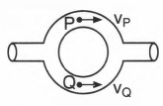a) 30 m/sec
b) 35 m/sec
c) None of these
d) 5 m/sec
11. In order to find time, the astronaut orbiting in an Earth satellite should use
a) a pendulum clock
b) a watch having main spring to keep it going
c) either a pendulum clock or a watch
d) neither a pendulum clock nor a watch
12. A man standing on a turn-table is rotating at a certain angular frequency with his arms outstretched. He suddenly folds his arms. If his moment of inertia with folded arms is 75% of that with outstretched arms, his rotational kinetic energy will
a) decrease by 33.3%
b) decrease by 25%
c) increase by 33.3%
d) increase by 25%
13. The ratio of the specific heats {tex}\frac{C_{p}}{C_{v}}=\gamma{/tex} in terms of degree of freedom (n) is given by
a) (1 + {tex}\frac{n}{3}{/tex})
b) (1 + {tex}\frac{1}{n}{/tex})
c) (1 + {tex}\frac{2}{n}{/tex})
d) (1 + {tex}\frac{n}{2}{/tex})
14. A tuning fork of frequency 580 Hz is employed to produce transverse waves on a long rope. The distance between the nearest crests is found to be 20 cm. The velocity of the wave is
a) 58 ms-1
b) 20 ms-1
c) 580 ms-1
d) 116 ms-1
15. An ideal heat engine exhausting heat at 77oC is to have a 30% efficiency. It must take heat at:
a) 673oC
b) 227oC
c) 327oC
d) 127oC
16. A space ship moves from the earth to the moon and back. The greatest energy required for the space ship is to overcome the difficulty in:
a) entering the moon’s lunar surface
b) take-off from lunar surface
c) entering the earth’s gravitational field
d) take-off from the earth’s field
17. Assertion (A): The perpendicular vector of {tex}(\hat{i}+\hat{j}+\hat{k}){/tex} is {tex}(\hat{i}-2 \hat{j}+\hat{k}){/tex}.
Reason (R): Two vectors are perpendicular if their dot product is equal to zero.

a) Both A and R are true and R is the correct explanation of A.
b) Both A and R are true but R is not the correct explanation of A.
c) A is true but R is false.
d) A is false but R is true.
18. Assertion: If the volume of a body remains unchanged, when subjected to tensile strain, the value of Poisson’s ratio is {tex}-\frac{1}{2}{/tex}.
Reason: Phosphor bronze has low Young’s modulus and higher rigidity modulus.

a) Assertion and reason both are correct statements and reason is correct explanation for assertion.
b) Assertion and reason both are correct statements but reason is not correct explanation for assertion.
c) Assertion is correct statement but reason is wrong statement.
d) Assertion is wrong statement but reason is correct statement.
19. Assertion (A): Pressure can be subtracted from pressure gradient.
Reason (R): Only like quantities can be added or subtracted from each other.

a) Both A and R are true and R is the correct explanation of A.
b) Both A and R are true but R is not the correct explanation of A.
c) A is true but R is false.
d) A is false but R is true.
20. ### Class 11 Physics Sample Paper Section B

21. In the case of Venus, the angle of maximum elongation is found to be approximately 47o. Determine the distance between Venus and the Sun rvs and the distance between Venus and the Earth.
22. A girl riding a bicycle along a straight road at a speed of 5 m s–1 throws a stone of mass 0.5 kg which has a speed of 15 ms–1 with respect to the ground along with her direction of motion. The mass of the girl and bicycle is 50 kg. Does the speed of the bicycle change after the stone is thrown? What is the change in speed, if so?
23. Draw graphs showing the variation of acceleration due to gravity with
1. height above the earth’s surface and
2. depth below the earth’s surface.

OR

Determine the speed with which the earth would have to rotate on its axis so that a Person on the equator would weigh {tex}\frac{3}{5}{/tex}th as much as at present. Take the equatorial radius as 6400 km.

24. Two rods of different materials but of equal cross-sections and lengths (1.0 m each) are joined to make a rod of length 2.0 m. The metal of one rod has a coefficient of linear thermal expansion 10-5 C-1 and Young’s modulus 3 {tex}\times{/tex} 1010 Nm-2. The other metal has the values 2 {tex}\times{/tex} 10-5 C-1 and 1010 Nm-2 respectively. How much pressure must be applied to the ends of the composite rod to prevent its expansion when the temperature is raised by 100o C?
25. Calculate the number of degrees of freedom of molecules of hydrogen in 1 cc of hydrogen gas at NTP.

OR

One mole of ideal monoatomic gas ({tex}\gamma{/tex} = 5/3) is mixed with one mole of diatomic gas ({tex}\gamma{/tex} = 7/5). What is {tex}\gamma{/tex} for the mixtures? Here {tex}\gamma{/tex} denotes the ratio of specific heat at constant pressure to that at constant volume.

26. A jet airplane travelling at the speed of 500 kmh-1 ejects its products of combustion at the speed of 1500 kmh-1 relative to the jet plane. What is the speed of the latter with respect to an observer on ground?
27. Block A of weight 100 N rests on a frictionless inclined plane of slope angle 30° (Fig.). A flexible cord attached to A passes over a frictionless pulley and is connected to block B of weight W. Find the weight W for which the system is in equilibrium.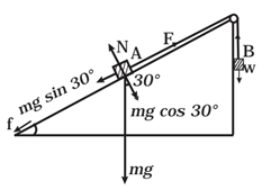28. ### Class 11 Physics Sample Paper Section C

29. Two rods A and B are of equal length. Each rod has its ends at temperatures T1 and T2. What is the condition that will ensure equal rates of flow of heat through the rods A and B?
30. A batsman deflects a ball by an angle of 45° without changing its initial speed which is equal to 54 km/h. What is the impulse imparted to the ball? (Mass of the ball is 0.15 kg.)
31. The flow rate of water from a tap of diameter 1.25 cm is 0.48 {tex}\frac{L}{min}{/tex}. The coefficient of viscosity of water is 10-3 Pa s. After some time the flow rate is increased to 3 L/min. Characterise the flow for both the flow rates.

OR

What is the pressure inside the drop of mercury of radius 3.00 mm at room temperature? Surface tension of mercury at that temperature (20°C) is 4.65 {tex}\times{/tex} 10-1 Nm-1. The atmospheric pressure is 1.01 {tex}\times{/tex} 105 Pa. Also give the excess pressure inside the drop.

32. The pattern of standing waves formed on a stretched string at two instants of time is shown in Figure. The velocity of two waves super-imposing to form stationary waves is 360 ms–1and their frequencies are 256 Hz.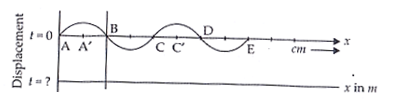1. Calculate the time at which the second curve is plotted.
2. Mark nodes and antinodes on the curve.
3. Calculate the distance between A′ and C ′.

OR

A SONAR system fixed in a submarine operates at a frequency 40.0 kHz. An enemy submarine moves towards the SONAR with a speed of 360 km h-1. What is the frequency of sound reflected by the submarine? Take the speed of sound in water to be 1450 ms-1.

33. A 20 cm thick layer of ice has been formed on the surface of a freshwater lake during extreme winter. The temperature of the air is -10oC. Find how long will it take for another 1mm layer of water to freeze? Thermal conductivity of ice = 2.1 W m-1 K-1, latent heat of fusion of water = 3.36 {tex}\times{/tex} 105 J kg-1 and density of ice = 103 kg m-3.
34. ### Class 11 Physics Sample Paper Section D

35. Fig. (a) shows a spring of force constant k clamped rigidly at one end and a mass m attached to its free end. A force F applied at the free end stretches the spring. Fig. (b) shows the same spring with both ends free and attached to a mass m at either end. Each end of the spring in Fig. (b) is stretched by the same force F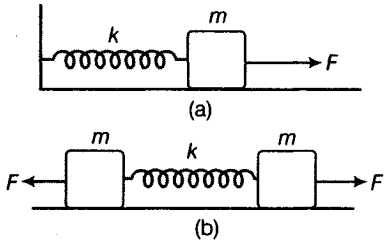1. What is the maximum extension of the spring in the two cases?
2. If the mass in Fig. (a) and the two masses in Fig. (b) are released, then what is the period of oscillation in each case?

OR

One end of a V-tube containing mercury is connected to a suction pump and the other end to atmosphere. The two arms of the tube are inclined to horizontal at an angle of 45° each. A small pressure difference is created between two columns when the suction pump is removed. Will the column of mercury in V-tube execute simple harmonic motion? Neglect capillary and viscous forces. Find the time period of oscillation.

36. A gun can fire shells with maximum speed {tex}\mathrm { v } _ { 0 }{/tex} and the maximum horizontal range that can be achieved is R ={tex}\frac { v _ { 0 } ^ { 2 } } { g }{/tex}. If a target farther away by distance {tex}\Delta x{/tex} (beyond R) has to be hit with the same gun as shown in the figure here, show that it could be achieved by raising the gun to a height at least h =  {tex}\Delta x \left[ 1 + \frac { \Delta x } { R } \right]{/tex}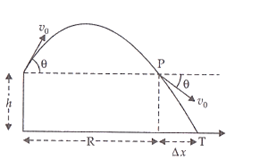[Hint: This problem can be approached in two different ways:

1. Refer to the diagram: target T is at the horizontal distance {tex}x = R + \Delta x{/tex} and below the point of projection y = – h.
2. From point P in the diagram: Projection at speed {tex}\mathrm { v } _ { 0 }{/tex} at an angle {tex}\theta{/tex} below horizontal with height h and horizontal range{tex}\Delta x{/tex}.]

OR

Establish the following vector inequalities:

1. {tex}|\vec{a}+\vec{b}| \leq|\vec{a}|+|\vec{b}|{/tex}
2. {tex}|\vec{a}-\vec{b}| \leq|\vec{a}|+|\vec{b}|{/tex}

When does the equality sign apply?

37. A car weighs 1800 kg. The distance between its front and back axles is 1.8 m. Its centre of gravity is 1.05 m behind the front axle. Determine the force exerted by the level ground on each front wheel and each back wheel.

OR

The two sides of a step ladder BA and CA are 1.6 m long and hinged at A. A rope DE, 0.5 m is tied half way up. A weight 40 kg is suspended from a point F, 1.2 m from B along the ladder BA. Assuming the floor to be frictionless and neglecting the weight of the ladder, find the tension in the rope and forces exerted by the floor on the ladder. (Take g = 9.8 m/s2)
(Hint: Consider the equilibrium of each side of the ladder separately.)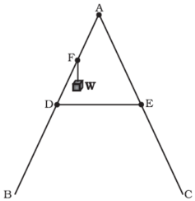To practice more questions & prepare well for exams, download myCBSEguide App. It provides complete study material for CBSE, NCERT, JEE (main), NEET-UG and NDA exams. Teachers can use Examin8 App to create similar papers with their own name and logo.

38. ### Class 11 Physics Sample Paper Section E

If the position of an object is continuously changing w.r.t. its surrounding, then it is said to be in the state of motion. Thus, motion can be defined as a change in position of an object with time. It is common to everything in the universe.
In the given figure, let P, Q and R represent the position of a car at different instants of time.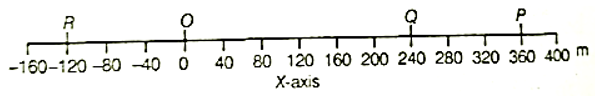1. With reference to the given figure, What are the position coordinates of points P and R?
2. Give any 2 differences between distance and displacement.
3. From the given figure, find the displacement of a car in moving from O to P and then to Q?
4. OR

If the car goes from O to P and returns back O, what will be the displacement of the car for whole journey?

There are many types of spring. Important among these are helical and spiral springs as shown in the figure.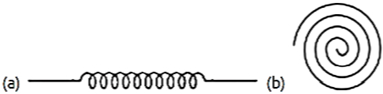Usually, we assume that the springs are massless. Therefore, work done is stored in the spring in the form of the elastic potential energy of the spring. Thus, the potential energy of a spring is the energy associated with the state of compression or expansion of an elastic spring.

1. When the potential energy of a spring may be considered as zero?
2. The ratio of spring constants of two springs is 2 : 3. What is the ratio of their potential energy, if they are stretched by the same force?
3. The potential energy of a spring increases by 15 J when stretched by 3 cm. If it is stretched by 4 cm, What will be the increase in potential energy?​​​​
4. OR

The potential energy of a spring when stretched through a distance x is 10 J. What is the amount of work done on the same spring to stretch it through an additional distance x?

Class 11 – Physics
Sample Paper – 01 (2022-23)

## Class 11 Physics Sample Paper Solution

1. ### Class 11 Physics Sample Paper Solution Section A

2. (b) Angular momentum
Explanation: Its value is approximately 6.626 {tex}\times {/tex} 10-34 joule-seconds (equivalent to units of angular momentum). Planck’s constant is fundamental to phenomena as the quantization of angular momentum and is used in Heisenberg’s Uncertainty Principle.
3. (a) Potential energy
Explanation: A stretched bow possesses potential energy. As soon as it is released, it shoots the arrow in the forward direction with a large velocity. The potential energy of the stretched bow gets converted into kinetic energy.
4. (d) {tex}m v^{3} /(4 \sqrt{2} g){/tex}
Explanation: {tex}L=m \frac{v}{\sqrt{2}} r_{\perp}{/tex}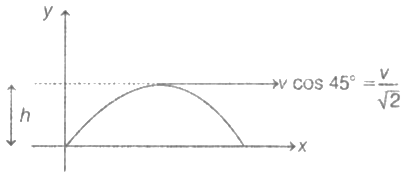Here, {tex}r_{\perp}=h=\frac{v^{2} \sin ^{2} 45^{\circ}}{2 g}=\frac{v^{2}}{4 g}{/tex}
{tex}\therefore \quad L=m\left(\frac{v}{\sqrt{2}}\right)\left(\frac{v^{2}}{4 g}\right)=\frac{m v^{3}}{4 \sqrt{2} g}{/tex}
5. (a) one-fourth for wire A as for wire B
Explanation: For same F, Y and L,
{tex}l \propto \frac{1}{A}{/tex}
{tex}\Rightarrow l \propto \frac{1}{r^{2}}{/tex}
{tex}\therefore \frac{l_{A}}{l_{B}}=\left(\frac{r_{B}}{r_{A}}\right)^{2}{/tex}
lA = 4lB
or lB{tex}\frac{l_{A}}{4}{/tex}
6. (b) g will decrease
Explanation: {tex}g=\frac{G M}{R^{2}}, v_{e}=\sqrt{\frac{2 G M}{R}}{/tex} and {tex}U=\frac{-G M m}{R}{/tex}
{tex}g \propto \frac{M}{R^{2}}, v_{e} \propto \sqrt{\frac{M}{R}}{/tex} and {tex}U \propto \frac{M}{R}{/tex}
If both mass and radius are increased by 0.5% then {tex}v_{e}{/tex} and U remains unchanged where as g decrease by 0.5%.
To practice more questions & prepare well for exams, download myCBSEguide App. It provides complete study material for CBSE, NCERT, JEE (main), NEET-UG and NDA exams. Teachers can use Examin8 App to create similar papers with their own name and logo.
7. (a) perfect and of constant mass and temperature
Explanation: To obey Boyle’s law gas must be ideal or perfect and its mass or no of moles must be the same and temperature must be constant
PV = nRT
if n = constant and T = constant then only PV = constant
8. (d) Internal energy and entropy are state functions
Explanation: Both the internal energy and entropy are unique functions of state only.
9. (c) decreases
Explanation: Here, vs = 0 and vL is negative, where vs is velocity of source and vL is velocity of listener (aeroplane)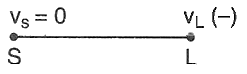If apparent frequency is v’ and v is actual frequency, then
{tex}v=\frac{v \ – \ \left(-v_{L}\right)}{v} v{/tex} = {tex}\frac{v \ + \ v_{L}}{v} v{/tex}
i.e., v’ > v
So, apparent frequency will increase, it means apparent wavelength will decrease.
10. (d) 5 m/sec
Explanation: Equation of continuity,
Rate = {tex}r=\frac{A}{3} V_{P}+\left(A-\frac{A}{3}\right) V_{Q}{/tex}
{tex}\therefore \quad V_{P}=2 V_{Q}=\frac{3 r}{A}=\frac{3 \times 0.1}{10^{-2}}=30 \mathrm{m} / \mathrm{sec}{/tex}
As VP=20 m/sec
Hence, V= 5 m/sec
11. (b) a watch having main spring to keep it going
Explanation: In the pendulum clock the time- period depends on the value of g, while in spring watch, the time period is independent of the value of g.
12. (c) increase by 33.3%
Explanation: Let I1 and {tex}\omega_1{/tex} be the moment of inertia and angular frequency respectively when his arms are outstretched and I2 and {tex}\omega_2{/tex} be their values when his arms are folded. Then, {tex}\mathrm{I}_{1} \omega_{1}=\mathrm{I}_{2} \omega_{2}{/tex}
Now, {tex}\mathrm{I}_{2}=\frac{3}{4} \mathrm{I}_{1}{/tex}. Hence {tex}\mathrm{I}_{1} \omega_{1}=\frac{3}{4} \mathrm{I}_{1} \omega_{2}{/tex}
or {tex}\omega_{2}=\frac{4}{3} \omega_{1}{/tex}
Initial K.E. is {tex}\mathrm{K}_{1}=\frac{1}{2} \mathrm{I}_{1} \omega_{1}{ }^{2}{/tex} and final K.E. is,
{tex}\mathrm{K}_{2}=\frac{1}{2} \mathrm{I}_{2} \omega_{2}^{2}=\frac{1}{2} \times \frac{3 \mathrm{I}_{1}}{4} \times\left(\frac{4 \omega_{1}}{3}\right)^{2}{/tex}
{tex}=\frac{4}{3}\left(\frac{1}{2} \mathrm{I}_{1} \omega_{1}{ }^{2}\right)=\frac{4}{3} \mathrm{~K}_{!}{/tex}
{tex}\therefore{/tex} Percentage increase in K.E.
{tex}\frac{\mathrm{K}_{2}-\mathrm{K}_{1}}{\mathrm{~K}_{1}} \times 100{/tex} = {tex}\frac{\left(\frac{4}{3} K_{1}-K_{1}\right)}{K_{1}} \times 100{/tex}
{tex}\frac{100}{3}{/tex} = 33.33 %
13. (c) (1 + {tex}\frac{2}{n}{/tex})
Explanation: (1 + {tex}\frac{2}{n}{/tex})
14. (d) 116 ms-1
Explanation: v = {tex}\nu{/tex}{tex}\lambda{/tex} = 580 {tex}\times{/tex} 0.20 ms-1 = 116 ms-1
15. (b) 227oC
Explanation: We know,
{tex}\eta=1-\frac{T_{1}}{T_{2}}{/tex}
{tex}\Longrightarrow \frac{30}{100}=1-\frac{77\ +\ 273}{T_{2}}{/tex}
{tex}\Longrightarrow \frac{350}{T_{2}}=1-\frac{3}{10}{/tex}
{tex}\Longrightarrow T_{2}=\frac{350 \ \times \ 10}{7}{/tex} = 500 K
Or, It must take heat at 500 – 273 = 227oC
16. (d) take-off from the earth’s field
Explanation: Focus acting on space ship are Gravitational pull due to Earth + Gravitational pull due to mass.
Now since the mass of Earth is greater that mass of moon.
Gravitational pull at Earth’s surface would be greater that at Moon’s surface.
Hence The space ship will require greatest energy to overcome the difficult in take off from earth’s field.
17. (a) Both A and R are true and R is the correct explanation of A.
Explanation: If the scalar product of two vectors are zero, then the vectors are perpendicular to each other. So
{tex}(\hat{i}+\hat{i}+\hat{k}) \cdot(\hat{i}-2 \hat{j}+\hat{k}){/tex} = {tex}\hat{i} \cdot \hat{i}-2(\hat{j} \cdot \hat{j})+\hat{k} \cdot \hat{k}{/tex} = 0
18. (c) Assertion is correct statement but reason is wrong statement.
Explanation: Let {tex}\Delta {r}{/tex} and {tex}\Delta {l}{/tex} be the change in radius and length of the wire.
{tex}\therefore{/tex} Change in volume {tex}=\pi r^{2} l-\pi(r-\Delta r)^{2}(l+\Delta l) {/tex}
{tex}=\pi r^{2} l-\pi\left(r^{2}+\Delta r^{2}-2 r \Delta r\right)(l+\Delta l){/tex}
{tex}\Delta V=\pi r^{2} l-\pi r^{2} l-\pi r^{2} \Delta l-2 \pi r \Delta r l{/tex}
(neglecting terms containing {tex}\Delta r^{2}{/tex} and {tex}\Delta r \Delta l{/tex})
Now, {tex}\Delta{/tex}V = 0 (given)
{tex}\therefore \quad 2 \pi r \Delta r l=\pi r^{2} \Delta l{/tex}
or {tex}\frac{\Delta r l}{r \Delta l}=\frac{1}{2} {/tex}
poisson’s ratio {tex}=\frac{\text { Lateral strain }}{\text { Longitudinal strain }}=-\frac{\Delta r/ r}{\Delta l/l}=-\frac{1}{2}{/tex}
Thus, statement A is correct. But statement B is wrong because Phosphor bronze has high Young’s modulus and low rigidity modulus.
19. (d) A is false but R is true.
Explanation: [Pressure gradient] = {tex}\left[\frac{d P}{d x}\right]=\frac{\left[\mathrm{ML}^{-1} \mathrm{~T}^{-2}\right]}{[\mathrm{L}]}=\left[\mathrm{ML}^{2} \mathrm{T}^{-2}\right]{/tex} which are not the dimensions of pressure, therefore pressure cannot be subtracted from a pressure gradient.
20. ### Class 11 Physics Sample Paper Solution Section B

21. Here angle of maximum elongation, {tex}\varepsilon{/tex} = 47o
Distance between Venus and the sun is
rvs = {tex}\sin \varepsilon . A U{/tex} = sin 47o {tex}\times{/tex} 1 AU
0.73 {tex}\times{/tex} 1.496 {tex}\times{/tex} 1011 m
Distance between Venus and the earth is
rve = {tex}\sin \varepsilon . A U{/tex} = sin 47o {tex}\times{/tex} 1 AU
= 0.68 {tex}\times{/tex} 1.496 {tex}\times{/tex} 1011 m = 1.02 {tex}\times{/tex} 1011 m
22. Girl and cycle  Body
m1 = 50 k g, m2 = 0.5 k g
u1 = 5 m/s forward  u2 = 5 m/s forward
v1 = ?, v2 = 15 m/s forward
According to the law of conservation of momentum.
Initial momentum (Girl, cycle, body)= Final momentum (cycle+Girl) and body
(m1 + m2) u1 = m1v1 + m2v2
( 50 + 0.5 ) {tex}\times{/tex} 5 = 50 {tex}\times{/tex} v1 + 0.5 {tex}\times{/tex} 15
50.5 {tex}\times{/tex} 5 – 7.5 = 50 v1
50 v1 = 252.5 – 7.5 = 245.0
{tex}v _ { 1 } = \frac { 245.0 } { 50 } = 4.9 \mathrm { m } / \mathrm { s }{/tex}
Hence, the speed of cycle and girl decreased by 5-4.9 = 0.1 m/s.
1. The value of g varies with height h as
{tex}g \propto \frac{1}{(R \ + \ h)^{2}}{/tex} or {tex}g \propto \frac{1}{r^{2}}{/tex}
Thus the graph of g versus r is the parabolic curve AB, as shown in Figure.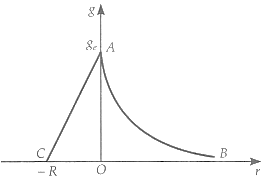2. The value of g varies with depth d as
g = {tex}g\left(1-\frac{d}{R}\right){/tex} i.e., g {tex}\propto{/tex} (R – d)
Thus the graph of g versus depth d is the straight line AC, as shown in Figure.

OR

Acceleration due to gravity at the equator is
{tex}g_e = g – r{/tex}{tex}\omega{/tex}2
{tex}mg_e = mg – mr{/tex}{tex}\omega{/tex}2
or {tex}\frac{3}{5}{/tex} {tex}mg = mg – mr{/tex}{tex}\omega{/tex} {tex}\left[\because m g_{e}=\frac{3}{5} m g\right]{/tex}
{tex}\therefore{/tex} {tex}\omega{/tex} = {tex}\sqrt{\frac{2 g}{5 R}}{/tex} = {tex}\sqrt{\frac{2 \times 9.8}{5 \times 6400 \times 10^{3}}}{/tex} {tex}= 7.8 \times 10^{-4} rad/s{/tex}

23. When the temperature is raised by 100o C, the extensions in the two rods are
{tex}\Delta l_{1}=\alpha_{1} l \Delta T{/tex} = 10-5 {tex}\times{/tex} 1.0 {tex}\times{/tex} 100 = 10-3 m
{tex}\Delta l_{2}=\alpha_{2} l \Delta T{/tex} = 2 {tex}\times{/tex} 10-5 {tex}\times{/tex} 1.0 {tex}\times{/tex} 100 = 2 {tex}\times{/tex} 10-3 m
Tensions produced in the rods are
T1{tex}Y_{1} A \cdot \frac{\Delta l_{1}}{l}=\frac{3 \times 10^{10} \times A \times 10^{-3}}{1.0}{/tex}
= 3 {tex}\times{/tex} 107 A newton
T2{tex}Y_{2} A \cdot \frac{\Delta l_{2}}{l}=\frac{10^{10} \times A \times 2 \times 10^{-3}}{1.0}{/tex}
= 2 {tex}\times{/tex} 107 A newton
where A = area of cross-section of each rod.
Total force needed to be applied at the ends to prevent expansion
= T1 + T2 = 5 {tex}\times{/tex} 107 A newton
{tex}\therefore{/tex} Pressure = {tex}\frac{\text { Force }}{\text { Area }}=\frac{5 \times 10^{7} A}{A}{/tex} = 5 {tex}\times{/tex} 107 Nm-2
24. Hydrogen molecule is diatomic so it has 3 translational degree of freedom and 2 rotational.
So degree of freedom in H2 molecule = 3 + 2 = 5
For number of molecule in 1 cc
22.4 lit = 22400 cc H2 gas at STP ahs = 6.023 {tex}\times{/tex} 1023
1 cc H2 gas at STP has {tex}=\frac{6.023}{22400} \times 10^{3}{/tex} molecule = 2.688 {tex}\times{/tex} 1019
So total degree of freedom = 5 {tex}\times{/tex} 2.688 {tex}\times{/tex} 1019{tex}=13.440 \times 10^{19}=1.344 \times 10^{20}{/tex}

OR

{tex}\gamma=\frac{C_{P}}{C_{V}}=\frac{C_{V}+R}{C_{V}}=1+\frac{R}{C_{V}} \text { or } C_{V}=\frac{R}{\gamma-1}{/tex}
For monoatomic gas, {tex}C_{V}=\frac{R}{5 / 3-1}=\frac{3}{2} R{/tex}
For diatomic gas {tex}C_{V}^{\prime}=\frac{R}{7 / 5-1}=\frac{5}{2} R{/tex}
CV (mixture) {tex}=\frac{n C_{V}+n^{\prime} C_{V}^{\prime}}{n+n^{\prime}}=\frac{1 \times \frac{3}{2} R+1 \times \frac{5}{2} R}{1+1}=2 R{/tex}
{tex}\gamma{/tex} (mixture) {tex}=1+\frac{R}{C_{V}(\text { mixture })}=1+\frac{R}{2 R}{/tex} = 1.5.

25. Speed of the jet airplane, vjet = 500 km/h
Relative speed of its products of combustion with respect to the plane,
vsmoke = – 1500 km/h
Speed of its products of combustion with respect to the ground = V”smoke
Relative speed of its products of combustion with respect to the airplane,
Vsmoke = V”smoke – Vjet
-1500 = V”smoke – 500
V”smoke = -1000 km/h
The negative sign indicates that the direction of its products of combustion is opposite to the direction of motion of the jet airplane.
26. In equilibrium there is no motion of blocks i.e, the forces should balance out.
For block B, the equation is:
F = Mg …(i)
For block A the equation is:
F – mg sin 30 = 0 …(ii)
Substituting the value of F from
Mg = mg sin 30
{tex}\Rightarrow{/tex} Mg=100 {tex}\times{/tex} 0.5 = 50 N
So the weight of the block should be 50 N
27. ### Class 11 Physics Sample Paper Solution Section C

28. By the formula of conduction of heat
{tex}\ Q = \frac { \mathrm { KA } \left( \theta _ { 1 } – \theta _ { 2 } \right) t } { x }{/tex}
Q = heat flow
K = co-efficient of thermal conductivity of substance
A = area of cross-sectional of body
{tex}\theta _ 1{/tex} = Temperature of hot surface of body
{tex}\theta _ 2{/tex} = Temperature of cold surface of body
X = distance between hot and cold surfaces
t = time for transfer of heat
For rod A :
{tex}\frac { \ Q _ { A } } { t } = \frac { K _ { A } \left( T _ { 1 } – T _ { 2 } \right) A _ { A } } { x }{/tex}………….(1)
And {tex}\frac { \ Q _ { B } } { t } = \frac { K _ { A } \left( T _ { 1 } – T _ { 2 } \right) A _ { B } } { x }{/tex}……………………(2)
For equal rates of flow, {tex}\frac { \theta _ { A } } { t } = \frac { \theta _ { B } } { t }{/tex}
So KA AA = KB AB
29. The ball struck by the bat is deflected back such that the total angle is 45o.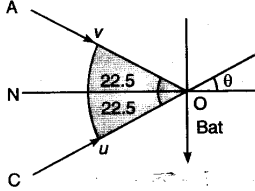Now, initial momentum of ball = mu cos {tex}\theta{/tex}
{tex}=\frac{0.15 \times 54 \times 1000 \times \cos 22.5}{3600}{/tex}
= 0.15 {tex}\times{/tex} 15 {tex}\times{/tex} 0.9239 along ON
Final momentum of ball = mucos {tex}\theta{/tex} along ON
Impulse = change in momentum
= mucos {tex}\theta{/tex} – (-mucos {tex}\theta{/tex})
= 2mucos {tex}\theta{/tex}
{tex}=2 \times 0.15 \times 15 \times 0.9239{/tex}
i.e., Impulse = 4.16 kg ms-1

30. Let the speed of the flow be v.
Given, diameter of tap = d = 1.25 cm
Volume of water flowing out per second.
Q = v {tex}\times \frac{\pi d^{2}}{4} \Rightarrow{/tex} v = {tex}\frac{4 Q}{d^{2} \pi}{/tex}
Estimate Reynold’s number, Re = {tex}\frac{4 \rho Q}{\pi d \eta}{/tex}
Q = 0.48 {tex}\frac{L}{min}{/tex} = 8 {tex}\times{/tex} 10-3 {tex}\frac{L}{s}{/tex} = 8 {tex}\times{/tex} 10-6 {tex}\frac{m^{3}}{s}{/tex}
{tex}R_e ={/tex} {tex}\frac{4 \times 10^{3} \times 8 \times 10^{-6}}{3.14 \times 1.25 \times 10^{-2} \times 10^{-3}}{/tex}
Re = 815 [i.e. below 1000, the flow is steady] After some time, when
Q = 3 {tex}\frac{L}{min}{/tex} = 5 {tex}\times{/tex} 10{tex}\frac{m^{3}}{s}{/tex}
Re{tex}\frac{4 \times 10^{3} \times 5 \times 10^{-5}}{314 \times 1.25 \times 10^{-2} \times 10^{-3}}{/tex} = 5095
{tex}\therefore{/tex} The flow will be turbulent.

OR

Radius of mercury drop r = 3.00 mm = 3 {tex}\times{/tex} 10-3m
surface tension of mercury S = 4.65 {tex}\times{/tex} 10-1 Nm-1
atmospheric pressure Patm = 1.01 {tex}\times{/tex} 105 pa
Total pressure (Ptotal) inside the mercury drop = excess pressure inside mercury + atmospheric pressure
equation for excess pressure inside mercury P = pi – p0 = {tex} \frac { 2 S } { r }{/tex}
thus Ptotal = P + Patm = {tex}\frac{2 S}{r}{/tex} + Patm
Ptotal {tex}\frac { 2 \times 4.65 \times 10 ^ { – 1 } } { 3 \times 10 ^ { – 3 } } {/tex}+ (1.01 {tex}\times{/tex} 105)
Ptotal = 1.0131 {tex}\times{/tex} 105 Pa
excess pressure P = {tex}\frac{2 S}{r}=\frac{2 \times 4.65 \times 10^{-1}}{3 \times 10^{-3}}{/tex}
P = 310 Pa

31. Given, frequency of the wave v = 256 Hz
Time period {tex}T=\frac{1}{v}=\frac{1}{256} s=3.9 \times 10^{-3}=s{/tex}

1. Time is taken to pass through mean position is
{tex}t=\frac{T}{4}=\frac{1}{40}=\frac{3.9 \times 10^{-3}}{4} s{/tex} {tex}=9.8 \times 10^{-4} s{/tex}
2. Nodes are A, B, C, D, E (i.e., zero displacements)
Antinodes are A’, C’ (i.e., maximum displacement)
3. It is clear from the diagram A’ and C’ are consecutive hence separation = wavelength ({tex}\lambda{/tex})
{tex}=\frac{v}{v}=\frac{360}{256}=1.41 \mathrm{m}{/tex}

OR

Operating frequency of the SONAR system, v = 40 kHz
Speed of the enemy submarine, ve = 360 km/h = 100 m/s
Speed of sound in water, v = 1450 m/s
The source is at rest and the observer (enemy submarine) is moving toward it. Hence, the apparent frequency (v’) received and reflected by the submarine is given by the relation:
{tex}\Rightarrow v ^ { \prime } = \left( \frac { v + v _ { e } } { v } \right) v{/tex}
{tex}= \left( \frac { 1450 + 100 } { 1450 } \right) \times 40 = 42.76 k H z{/tex}
The frequency (v”) received by the enemy submarine is given by the relation:
{tex}\Rightarrow v ^ { \prime \prime } = \left( \frac { v } { v + v _ { s } } \right) v'{/tex}
Where, vs = 100m/s
So, frequency of sound received is:
{tex}\Rightarrow v ^ { \prime \prime } = \left( \frac { 1450 } { 1450 – 100 } \right) \times{/tex} 42.76 = 45.93 kHz

32. Given: Thickness of ice layer formed, l = 20 cm = 0.2 m,
Temperature just below ice layer (in the lake) T1 = 0oC,
Temperature of air (above ice layer) T2 = -10oC,
Thermal conductivity of ice K = 2.1 W m-1K-1,
Latent heat of fusion of water Lf = 3.36 {tex}\times{/tex} 105 J kg-1 and ice density {tex}\rho{/tex} = 103 kg m-3.
Let A be the surface area of the lake that takes time t for the formation of ice layer by {tex}\Delta l=1 \mathrm{mm}=10^{-3} \mathrm{m}{/tex}
{tex}\therefore{/tex} Mass of water formed in time t, m = A.{tex}\Delta l . \rho=A \times 10^{-3} \times 10^{3}{/tex} = A kg
From the heat energy relation, H = {tex}\frac{\Delta Q}{\Delta t}{/tex} = {tex}\frac{K A\left(T_{1}-T_{2}\right)}{l}{/tex}, we have
{tex}\frac{m L_{f}}{t}{/tex} = {tex}\frac{A L_{f}}{t}{/tex} = {tex}\frac{K A\left(T_{1}-T_{2}\right)}{l}{/tex}
or t = {tex}\frac{L_{f} \cdot l}{K\left(T_{1}-T_{2}\right)}{/tex} = {tex}\frac{3.36 \times 10^{5} \times 0.2}{2.1 \times[0-(-10)]}{/tex} = {tex}\frac{3.36 \times 10^{5} \times 0.2}{2.1 \times 10}{/tex}
= 3200 s = 53 min 20 s.
So it would take 53 min 20 sec to form the layer of ice.
33. ### Class 11 Physics Sample Paper Solution Section D

1. For Case (a), as we know that the restoring force is given by , F = -kx {tex}\Rightarrow{/tex} |F| = kx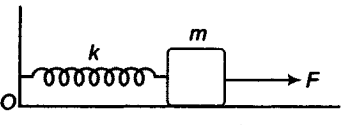So, x = {tex}\frac{F}{k}{/tex}
for the Case (b)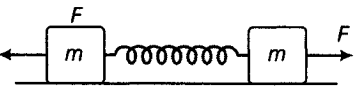If x’ is the extension in the spring, then drawing free body diagram of either mass (as the system under applied force is under equilibrium).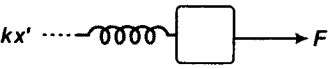kx’ = F
{tex}\therefore x ^ { \prime } = \frac { F } { k }{/tex}
In both the cases, extension is the same{tex}\left( \frac { F } { k } \right){/tex}.
2. The period of oscillation in case(a)
As, restoring force(F) is given by  = -kx
where, x = is the given extension
But from Newton’s 2nd law of motion we know that, F = ma
{tex}\therefore m a = – k x \Rightarrow a = – \left( \frac { k } { m } \right) x{/tex}…….(i)
{tex}a \propto – x{/tex}
On comparing eq.(i) with a = –{tex}\omega{/tex}2x, we get
{tex}\omega = \sqrt { \frac { k } { m } }{/tex}(angular frequency or velocity of the motion)
Period of oscillations is given by, {tex}T = \frac { 2 \pi } { \omega } = 2 \pi \sqrt { \frac { m } { k } }{/tex}
Case (b)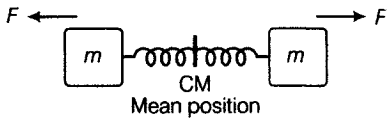The system is divided into two similar systems with spring divided in two equal halves, forming spring constant hence,
k’ = 2k
Hence, force is , F = – k’x
Putting k’ = 2k (on cutting a spring in two halves, its k doubles)
F = -2kx
But from Newton’s law of motion, force is given by F = ma
{tex}\therefore{/tex}ma = -2kx
{tex}\Rightarrow a = – \left( \frac { 2 k } { m } \right) x{/tex}   . . . . . (ii)
On comparing Eq.(ii) with a = –{tex}\omega{/tex}2x, we get angular frequency or velocity is,
{tex}\omega = \sqrt { \frac { 2 k } { m } }{/tex}
Hence the required period of oscillation of the given question,
{tex}T = \frac { 2 \pi } { \omega } = 2 \pi \sqrt { \frac { m } { 2 k } }{/tex}

OR

L et the liquid column in both arms of the V-tube were at {tex}h _ { 0 }{/tex} heights initially. Now due to pressure difference the liquid columns in A arm is pressed by {tex}x{/tex} and in arm B is liftted by {tex}x{/tex} (so difference in vertical height between two levels {tex}= 2 x{/tex}) Consider an element of liquid of height dx inside the tube.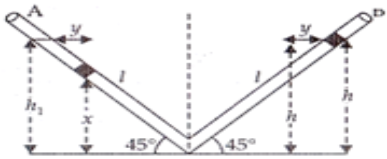Then its dm = volume {tex}\times{/tex} density =  A .dx{tex}\rho{/tex}(where, A =area of cross-section of tube, {tex}\rho{/tex} = density of the liquid inside the tube)
Potential energy of the right arm with {tex}d m{/tex} elementary mass column = (dm) gh
Potential energy of {tex}d m{/tex} elementary mass in left arm column {tex}= A \rho g x d x{/tex} (putting the value of dm = {tex}A .dx.\rho{/tex} and h = x)
{tex}\therefore{/tex}Total potential energy in left column {tex}= \int _ { 0 } ^ { h_1 } A \rho g x d x = A \rho g \left[ \frac { x ^ { 2 } } { 2 } \right] _ { 0 } ^ { h_1 }{/tex}
{tex}= A \rho g \frac { h_1 ^ { 2 } } { 2 }{/tex}
From above given figure  {tex}\sin 45 ^ { \circ } = \frac { h _ { 1 } } { l }{/tex}  {tex}\therefore h _ { 1 } = h _ { 2 } = l \sin 45 ^ { \circ } = \frac { l } { \sqrt { 2 } }{/tex}
{tex}\therefore h _ { 1 } ^ { 2 } = h _ { 2 } ^ { 2 } = \frac { l ^ { 2 } } { 2 }{/tex}
{tex}\therefore{/tex} Potential energy in the left column = {tex}A \rho g \frac { l ^ { 2 } } { 4 }{/tex}
Similarly potential energy in right column{tex}= A \rho g \frac { l ^ { 2 } } { 4 }{/tex}
{tex}\therefore{/tex}  Total potential energy {tex}= A \rho g \frac { l ^ { 2 } } { 4 } + A \rho g \frac { l ^ { 2 } } { 4 } = \frac { A \rho g l ^ { 2 } } { 2 }{/tex}
Due to pressure difference, left element moves towards right side by ‘y’ units and the same element rises in the right arm by ‘y’ units.
Then the liquid column length in the left arm becomes by decreasing = ( l – y )
And the liquid column length in the right arm becomes by increasing = ( l + y )
Now decreased potential energy of liquid column in the left arm {tex}= A \rho g ( l – y ) ^ { 2 } \sin ^ { 2 } 45 ^ { \circ }{/tex}
Similarly increased potential energy of liquid column in the right arm {tex}= A \rho g ( l + y ) ^ { 2 } \sin ^ { 2 } 45 ^ { \circ }{/tex}
{tex}\therefore{/tex}  Total potential energy due to two liquid columns in the left and right arm respectively {tex}= A \rho g \left( \frac { 1 } { \sqrt { 2 } } \right) ^ { 2 } \left[ ( l – y ) ^ { 2 } + ( l + y ) ^ { 2 } \right]{/tex}
Final potential energy due to difference in liquid columns in the two arms,
{tex}= \frac { A \rho g } { 2 } \left[ l ^ { 2 } + y ^ { 2 } – 2 l y + l ^ { 2 } + y ^ { 2 } + 2 l y \right]{/tex}
{tex}\therefore {/tex} Final potential energy {tex}= \frac { A \rho g } { 2 } \left( 2 l ^ { 2 } + 2 y ^ { 2 } \right){/tex}
Now change in potential energy = Final potential energy due to liquid columns in the two arms– Initial potential energy due to liquid columns in the two arms
{tex}= \frac { A \rho g } { 2 } \left( 2 l ^ { 2 } + 2 y ^ { 2 } \right) – \frac { A \rho g l ^ { 2 } } { 2 }{/tex}
{tex}= \frac { A \rho g } { 2 } \left[ 2 l ^ { 2 } + 2 y ^ { 2 } – l ^ { 2 } \right]{/tex}
{tex}\therefore {/tex} Change in potential energy = {tex}\frac { A \rho g } { 2 } \left( l ^ { 2 } + 2 y ^ { 2 } \right){/tex}
If change in velocity (v) of total liquid column be v then change in kinetic energy,
{tex}\Delta K E = \frac { 1 } { 2 } m v ^ { 2 }{/tex}
Again m = volume {tex}\times{/tex} density =  (A.2l ) {tex}\rho{/tex}
{tex}\therefore \Delta K E = \frac { 1 } { 2 } ( A 2 l \rho ) v ^ { 2 } = A \rho l v ^ { 2 }{/tex}
{tex}\therefore{/tex} Change in Total energy = change in potential energy + change in kinetic energy {tex}= \frac { A \rho g } { 2 } \left( l ^ { 2 } + 2 y ^ { 2 } \right) + A \rho l v ^ { 2 }{/tex}
Again, from the law of conservation of energy, total change in energy {tex}\Delta P E + \Delta K E = 0{/tex}
{tex}\therefore \frac { A \rho g } { 2 } \left[ l ^ { 2 } + 2 y ^ { 2 } \right] + A \rho l v ^ { 2 } = 0{/tex}
{tex}\therefore \frac { A \rho } { 2 } \left[ g \left( l ^ { 2 } + 2 y ^ { 2 } \right) + 2 l v ^ { 2 } \right] = 0{/tex}
{tex}\because \frac { A \rho } { 2 } \neq 0{/tex}
{tex}\therefore g \left( l ^ { 2 } + 2 y ^ { 2 } \right) + 2 l v ^ { 2 } = 0{/tex}
Differentiating on both sides of the above equation with respect to time,t we get {tex}g \left[ 0 + 2 \times 2 y \frac { d y } { d t } \right] + 2 l .2 v \cdot \frac { d v } { d t } = 0{/tex}
{tex}\therefore 4 g y \frac { d y } { d t } + 4 v l \frac { d ^ { 2 } y } { d t ^ { 2 } } = 0{/tex} {tex}\left[ \because a = \frac { d v } { d t } = \frac { d ^ { 2 } y } { d t ^ { 2 } } \right]{/tex}
{tex}\Rightarrow 4 g y \cdot v + 4 v l \frac { d ^ { 2 } y } { d t ^ { 2 } } = 0 \Rightarrow 4 v \left[ g y + l \cdot \frac { d ^ { 2 } y } { d t ^ { 2 } } \right] = 0{/tex}
{tex}\Rightarrow \frac { d ^ { 2 } y } { d t ^ { 2 } } + \frac { g } { l } y = 0 \quad \because 4 v \neq 0{/tex}….(i)
It is the equation of a simple harmonic motion and can be compared with the standard equation of a simple harmonic motion i.e. {tex}\frac { d ^ { 2 } y } { d t ^ { 2 } } + \omega ^ { 2 } y = 0{/tex}…..(ii) [{tex}\omega{/tex} is the angular acceleration or angular frequency of the particle executing simple harmonic motion] Comparing the above two equations (i) and (ii) we get, {tex}\therefore { \omega ^ { 2 } } = \frac { g } { l }{/tex} ​​​​​​​
{tex}\therefore \frac { 2 \pi } { T } = \sqrt { \frac { g } { l } } \Rightarrow T = 2 \pi \sqrt { \frac { l } { g } }{/tex} [{tex}\because \omega = \frac {2π}{T}{/tex}, T being time period of the simple harmonic motion]

34. Maximum range of projectile{tex}R = \frac{{{V_0}^2}}{g}{/tex} ………(i)
We know, maximum range is achieved at {tex}θ = 45°​​​​​​​{/tex}
Let the gun is raised to a height h from the horizontal level of target T, so that the projectile can hit the target T.
Total range of projectile must be {tex}R _ { \text {total} } = R + \Delta x{/tex}
Horizontal component of velocity at {tex}A = v _ { 0 } \cos ( \theta ){/tex}
As A and P are on the same level, the magnitude of velocity will be the same at A and P.
​​​​​But the direction of velocity will be below horizontal,
So horizontal velocity at P, {tex}v _ { x } = – v _ { 0 } \cos ( \theta ){/tex}
and vertical velocity at P, {tex}v _ { y } = v _ { 0 } \sin ( \theta ){/tex}
Now {tex}h = u t + \frac { 1 } { 2 } a t ^ { 2 }{/tex}
{tex}h = – v _ { 0 } \sin \theta ( t ) + \frac { 1 } { 2 } g t ^ { 2 }{/tex}…….(ii)
Consider horizontal motion from A to T , distance {tex}( R + \Delta x ) = v _ { 0 }{/tex}cos {tex}\theta . t{/tex}
{tex}t = \frac { R + \Delta x } { v _ { 0 } \cos \theta }{/tex}
Substitute t in (ii) we get
{tex}h = – v _ { 0 } \sin \theta \left[ \frac { R + \Delta x } { \nu _ { 0 } \cos \theta } \right] + \frac { 1 } { 2 } g \frac { ( R + \Delta x ) ^ { 2 } } { v _ { 0 } ^ { 2 } \cos ^ { 2 } \theta }{/tex}
{tex}h = – ( R + \Delta x ) + \frac { 1 } { R } \left( R ^ { 2 } + \Delta x ^ { 2 } + 2 R \Delta x \right) \left[ \because \frac { g } { v _ { 0 } ^ { 2 } } = \frac { 1 } { R } \right]{/tex}
h  {tex}= – R – \Delta x + R + \frac { \Delta x ^ { 2 } } { R } + 2 \Delta x = \Delta x + \frac { \Delta x ^ { 2 } } { R }{/tex}
{tex}h = \Delta x \left[ 1 + \frac { \Delta x } { R } \right]{/tex}
Hence Proved.

OR

1. If {tex}\theta{/tex} be the angle between {tex}\vec{a}{/tex} and {tex}\vec{b}{/tex}, then
{tex}|\vec{a}+\vec{b}|=\sqrt{|\vec{a}|^{2}+|\vec{b}|^{2}+2|\vec{a}||\vec{b}| \cos \theta}{/tex}
Now {tex}|\vec{a}+\vec{b}|{/tex} will be maximum when
cos {tex}\theta{/tex} = 1 or {tex}\theta{/tex} = 0
{tex}\therefore |\vec{a}+\vec{b}|_{\max }{/tex} {tex}=\sqrt{|\vec{a}|^{2}+|\vec{b}|^{2}+2|\vec{a}||\vec{b}| \cos 0^{\circ}}{/tex}
{tex}=\sqrt{(|\vec{a}|+|\vec{b}|)^{2}}=|\vec{a}|+|\vec{b}|{/tex}
Hence {tex}|\vec{a}+\vec{b}| \leq|\vec{a}|+|\vec{b}|{/tex}
The equality sign in applicable when {tex}\theta{/tex} = 0o i.e., when {tex}\vec{a}{/tex} and {tex}\vec{b}{/tex} are in the same direction.
2. If {tex}\theta{/tex} is the angle between {tex}\vec{a}{/tex} and {tex}\vec{b}{/tex}, then the angle between {tex}\vec{a}{/tex} and {tex}-\vec{b}{/tex} will be (180o{tex}\theta{/tex}), as shown in figure.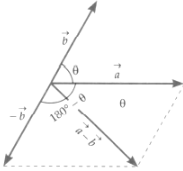{tex}=\sqrt{|\vec{a}|^{2}+|-\vec{b}|^{2}+2|\vec{a}||-\vec{b}| \cos \left(180^{\circ}-\theta\right)}{/tex}
{tex}=\sqrt{|\vec{a}|^{2}+|\vec{b}|^{2}-2|\vec{a}||\vec{b}| \cos \theta}{/tex}
{tex}\left[\because|-\vec{b}|=|\vec{b}|, \cos \left(180^{\circ}-\theta\right)=-\cos \theta\right]{/tex}
{tex}|\vec{a}-\vec{b}|{/tex} will be maximum when cos {tex}\theta{/tex} = -1 or {tex}\theta{/tex} = 180o
{tex}\therefore|\vec{a}-\vec{b}|_{\max }{/tex} {tex}=\sqrt{|\vec{a}|^{2}+|\vec{b}|^{2}-2|\vec{a}||\vec{b}| \cos 180^{\circ}}{/tex}
{tex}=\sqrt{(|\vec{a}|+|\vec{b}|)^{2}}=|\vec{a}|+|\vec{b}|{/tex}
Hence {tex}|\vec{a}-\vec{b}| \leq|\vec{a}|+|\vec{b}|{/tex}
The equality sign is applicable when {tex}\theta{/tex} = 180°

To practice more questions & prepare well for exams, download myCBSEguide App. It provides complete study material for CBSE, NCERT, JEE (main), NEET-UG and NDA exams. Teachers can use Examin8 App to create similar papers with their own name and logo.

35. Weight of car = 1800 Kg
Distance of COG from front axle = 1.05 m
Distance of COG from back axle = 1.8 – 1.05 = 0.75 m
Vertical forces are balanced ,
So,At translational equilibrium:
R1 + R2 = mg
R1 + R2 = 1800 {tex}\times{/tex} 9.8 = 17640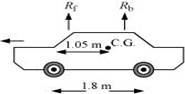R1 and R2 are the forces exerted by the level ground on the front and back wheels respectively.
Angular momentum about centre of gravity is zero.
So,
R1(1.05) = R2(1.8 – 1.05)
{tex}R _ { 1 } \times 1.05 = R _ { 2} \times 0.75{/tex}
{tex}\frac { R _ { 1 } } { R _ { 2 } } = \frac { 0.75 } { 1.05 } = \frac { 5 } { 7 }{/tex}
{tex}\frac { R _ { 1 } } { R _ { 2 } } = \frac { 7 } { 5 }{/tex}
R1 = 1.4 R2 …..(ii)
Solving equations (i) and (ii), we get:
1.4 R2 + R2 = 17640
{tex}R _ { 2 } = \frac { 17640 } { 2.4 } = 7350 \mathrm { N }{/tex}
{tex}\therefore{/tex} R1 = 17640 – 7350 = 10290 N
Therefore, the force exerted on each front wheel {tex}=\frac { R_1 } { 2 }= \frac { 7350 } { 2 } = 3675 N{/tex}, and
The force exerted on each back wheel {tex}= \frac { R_2 } { 2 }=\frac { 10290 } { 2 } = 5145 N{/tex}

OR

The given situation can be shown as: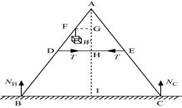NB = Force exerted on the ladder by the floor point B
NC = Force exerted on the ladder by the floor point C
T = Tension in the rope
BA = CA = 1.6 m
DE = 0. 5 m
BF = 1.2 m
Mass of the weight, m = 40 kg
Draw a perpendicular from A on the floor BC. This intersects DE at mid-point H.
{tex} \Delta{/tex}ABI and {tex} \Delta{/tex}AIC are similar
{tex}\therefore{/tex} BI = IC
Hence, I is the mid-point of BC.
DE || BC
BC = 2 {tex}\times{/tex} DE = 1 m
AF = BA – BF = 0.4 m …(i)
D is the mid-point of AB.
Hence, we can write:
{tex}A D = \frac { 1 } { 2 } \times B A = 0.8 \mathrm { m }{/tex} ….(ii)
Using equations (i) and (ii), we get:
FE = 0.4 m
Hence, F is the mid-point of AD.
FG || DH and F is the mid-point of AD. Hence, G will also be the mid-point of AH.
{tex}\therefore \frac { F G } { D H } = \frac { A F } { A D }{/tex}
{tex}\frac { F G } { D H } = \frac { 0.4 } { 0.8 } = \frac { 1 } { 2 }{/tex}
{tex}F G = \frac { 1 } { 2 } D H{/tex}
{tex}= \frac { 1 } { 2 } \times 0.25 = 0.125{/tex}
{tex}A H = \sqrt { A D ^ { 2 } – D H ^ { 2 } }{/tex}
{tex}= \sqrt { ( 0.8 ) ^ { 2 } – ( 0.25 ) ^ { 2 } } = 0.76 m{/tex}
For translational equilibrium of the ladder, the upward force should be equal to the downward force.
NC + NB = mg = 392 …(iii)
For rotational equilibrium of the ladder, the net moment about A is:
{tex}- N _ { B } \times B I + m g \times F G + N _ { c } \times C I + T \times A G – T \times A G = 0{/tex}
{tex}- N _ { B } \times 0.5 + 40 \times 9.8 \times 0.125 + N _ { c } \times ( 0.5 ) = 0{/tex}
{tex}\left( N _ { c } – N _ { B } \right) \times 0.5 = 49{/tex}
Nc – NB = 98
Adding equations (iii) and (iv), we get:
NC = 245 N
NB = 147 N
For rotational equilibrium of the side AB, consider the moment about A.
{tex}- N _ { B } \times B I + m g \times F G + T \times A G = 0{/tex}
{tex}- 245 \times 0.5 + 40 + 9.8 \times 0.125 + T \times 0.76 = 0{/tex}
0.76 T = 122.5 – 49
{tex}\therefore{/tex} T = 96.7 N
Hence, tension in the given question will be 96.7 N from the above calculation.

36. ### Class 11 Physics Sample Paper Solution Section E

1. The position coordinates of point P = (+360, 0, 0) and point R = (-120, 0, 0)
2. Distance can not be negative while displacement of a body can be negative or zero.
Distance is a scalar quantity while displacement is a vector quantity.
3. Displacement from O to P =  240 m
Displacement from P to Q =  240-300 = -60 m
Total displacement = 240-60 = 180 m
4. OR

As the initial and final position are same, so displacement will be 0 m.

1. It may be considered as zero, when the spring is in normal position.
2. {tex}\frac{E_1}{E_2}=\frac{k_1 x_1^2}{k_2 x_2^2}{/tex}
here x1 = x2
so {tex}\frac{E_1}{E_2}=\frac{k_1}{k_2}=\frac{2}{3}{/tex}​​​​​​​
3. ​​​​​​{tex}\frac{E_1}{E_2}=\frac{k\;x_1^2}{k\;x_2^2}{/tex}
here x1 = 3 cm and x2 = 4 cm
so {tex}\frac{E_1}{E_2} =\frac{x_1^2}{x_2^2} =\frac9{16}{/tex}
here E1 = 15 J
so E2 = {tex}\frac{16}9\times E_1{/tex} {tex}= \frac{16}9\times15{/tex} = 26.7 J
4. OR

​​​​​{tex}\frac{E_1}{E_2}=\frac{k x_1^2}{k x_2^2}{/tex}
{tex}E=\frac{1}{2} k x^2{/tex}
if distance = x, E1 = {tex}\frac12{/tex}​ kx2 = 10 J
on increasing the distance x more
E2 = {tex}\frac{1}{2} k(2 x)^2{/tex} = 2kx2
so increase in potential energy = E2 – E1 = (2 – {tex}\frac12{/tex}) KX2
{tex}=\frac{3}{2} K x^2=3 \times \frac{1}{2} k x^2{/tex} = 3 {tex}\times{/tex} 10 = 30 Joule
so work required = 30 – 10 = 20 Joule

## CBSE Sample Papers for Class 11

To download sample paper for class 11 Physics, Chemistry, Biology, History, Political Science, Economics, Geography, Computer Science, Home Science, Accountancy, Business Studies and Home Science; do check myCBSEguide app or website. myCBSEguide provides sample papers with solution, test papers for chapter-wise practice, NCERT solutions, NCERT Exemplar solutions, quick revision notes for ready reference, CBSE guess papers and CBSE important question papers. Sample Paper all are made available through the best app for CBSE students and myCBSEguide website.### Test Generator

Create question paper PDF and online tests with your own name & logo in minutes.### myCBSEguide

Question Bank, Mock Tests, Exam Papers, NCERT Solutions, Sample Papers, Notes

### 1 thought on “CBSE Sample Papers Class 11 Physics 2023”

1. Cbse sample paper helps me lot to scoreing excellent marks in physics.